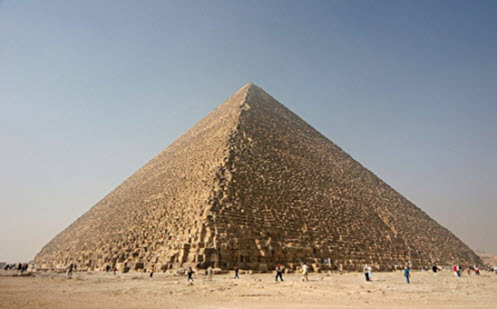Go to website

# Pyramids

Students begin by becoming aware of the features of a pyramid, determining the perpendicular height using Pythagoras' theorem as well as calculating the area, perimeter, volume and capacity of pyramids. Students then work with circles calculating the radius and diameter of the shape.

Year level(s) Year 9
Audience Teacher
Purpose Content knowledge, Evidence-based approaches, Student task, Teaching resource, Teaching strategies
Teaching strategies and pedagogical approaches Collaborative learning, Explicit teaching, Setting goals, Structuring lessons, Worked examples
Keywords circumference, radius, diameter, area, height, perimeter, volume, capacity, perpendicular height, Pythogoras theorem

## Curriculum alignment

Curriculum connections English, Numeracy
Strand and focus Measurement, Geometry, Build understanding, Apply understanding
Topics Angles and geometric reasoning
AC: Mathematics content descriptions
ACMMG222 Investigate Pythagoras’ Theorem and its application to solving simple problems involving right angled triangles
National numeracy learning progression Understanding geometric properties
Understanding units of measurement - UuM7